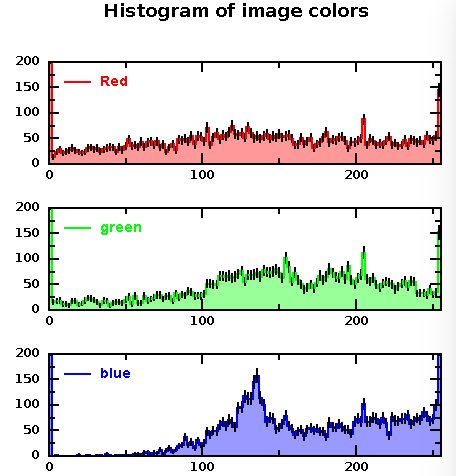# DMelt:Image/1 Image Manipulation

(diff) ← Older revision | Latest revision (diff) | Newer revision → (diff)
Limitted access. First login to DataMelt if you are a full DataMelt member. Then login to HandWiki as a user.

# Image manipulation using Java scripting

## Viewing an image

Run this code to display an image file:

from jhplot import *
IView("a.png")

This tool is designed for image viewing, without editing option.

## Grabbing image data

Images can be manipulated with DataMelt using several ways. Try to open an image using the ImageJ editor as [Tools]->[ImageJ]. Using the ImageJ GUI, open any file. Once opened, you can do verious manipulations.

You can also work with images without GUI. You can use Java API of this packageIJ package. Here is the example how to import the main class:

>>> from ij import IJ
>>> print  dir(IJ)

This prints all methods associated with the classij.IJ class.

from ij import *
imp = IJ.openImage("http://jwork.org/dmelt/logo.png") # grab an image from URL or a file
imp.show()               # display it in a new window

The following methods can be used to access information on image:

pixels = imp.getProcessor().getPixels() # get array of bytes
width = imp.getWidth()                 # get width (in Nr pixels)
high=imp.getHight()                     # get height
r = imp.getProcessor().getRoi()   # java.awt.Rectangle of this image

print "number of pixels:", imp.width * imp.height
print "number of slices:", imp.getNSlices()
print "number of channels:", imp.getNChannels()
types = {ImagePlus.COLOR_RGB : "RGB",
ImagePlus.GRAY8 : "8-bit",
ImagePlus.GRAY16 : "16-bit",
ImagePlus.GRAY32 : "32-bit",
ImagePlus.COLOR_256 : "8-bit color"}

print "image type:", types[imp.type]

## Getting pixel arrays and getting the image back

from ij import *
from ij.process import *
imp = IJ.openImage("https://datamelt.org/logo.png")
pix = imp.getProcessor().convertToRGB().getPixels() # RGB version of this image as a ColorProcessor
print pix

The one-dimensional array has the length equal to width time hight, i.e. it is equal to

imp.getWidth()*imp.getHeight()

ColorProcessors return the pixel array as an int[]. The values of the three color components (integer values 0-255) are packed into one int variable. They can be accessed as follows:

red =    (pix[i] & 0xff0000)>>16
green =  (pix[i] & 0x00ff00)>>8
blue  =  (pix[i] & 0x0000ff)

Here red,green,blue have values from 0 - 255. If you know (red,green,blue), then you can generate a new arrays as:

pix[i]= ((red & 0xff)<<16)+((green & 0xff)<<8) + (blue & 0xff)

Let us print (red,green,color) of the current image:

w=imp.getWidth()
h=imp.getHeight()
for  i in range(h):
offset = i*w
for  j in range(w):
c=pixRB[offset+j]
red =    (c & 0xff0000)>>16
green =  (c & 0x00ff00)>>8
blue  =  (c & 0x0000ff)
print red,green,blue

You can use a useful classjhplot.utils.ColorPixel which performs conversions between pixel colors and the usual RGB integer values (0-255).

The image can be created again from the array "pix" as:

img1=ImagePlus("New image", ColorProcessor(imp.width, imp.height, pix))
img1.show()

Similarly, pixels can be extracted using 32-bit float version of this image as a FloatProcessor. In this case the image will be converted to gray color representation using the formula g=(r+g+b)/3 and returns it as a float.

from ij import *
from ij.process import *
imp = IJ.openImage("https://datamelt.org/logo_dmelt.png")
pix = imp.getProcessor().convertToFloat().getPixels() # Graystyle image using 32-bit float representation
img1=ImagePlus("New image from pixels", FloatProcessor(imp.width, imp.height, pix, None))
img1.show()

Analysis and manipulation using Jython scripting will be discussed below.ere we will show how to create a random image using Jython

from ij import *
from ij.process import *
from java.util import Random
imp = IJ.createImage("A Random Image", "8-bit", 200, 200, 1)
Random().nextBytes(imp.getProcessor().getPixels())
imp.show()

This will show a frame 200x200 with an image containing random points. The API of ImageJ (and this is what is used by DataMelt) is very powerful for performing a detailed analysis of images, transformation and manipulation.

## Image analysis

As example of the above methods, below we show how to make histograms of image colors, extracting a vector with pixel colors, converting it to 3 arrays for red, green and blue and building histograms using DataMelt API. To speed-up the code, we usejhplot.utils.ColorPixel which converts ImageJ pixel colors into the usual 3 integer RGB values (0-255). This class also helps integrate image manipulation methods into the API of dmelt.

The output shows frequencies of each color in form of histograms (axis range is 0-255).One can use many statistical methods to study the image as described in section DMelt:Statistics/1_General_Topics. For example, print all statistical characteristics for each color as:

print rgb.getStatString()     # print detailed characteristics for red
print rgb.getStatString()     # print detailed characteristics for blue
print rgb.getStatString()     # print detailed characteristics for green